# The Keyword Of In Math

in STEMGeeks11 months ago

Hello there. In this education & mathematics post, I would like to cover the keyword of. There are actually quite a few cases where the keyword of is used in the math setting. For the most part the keyword of means to take a portion of something. This post covers the different cases with this keyword.Pixabay Image Source

## Topics

• Taking A Part/Fraction Of A Whole
• Multiplying Fractions Case
• Percentages
• Sample Of A Whole

## Taking A Part/Fraction Of A Whole

The keyword of means to take a part or fraction of a whole. As one example one half of 50 is 25. The number fifty is the whole, the number 25 is the part of a whole. The half represents the fraction of the whole.

Example One

Another example would be one quarter of 1000. Taking one quarter of 1000 means to divide 1000 by 4 to obtain 250. One thousand is the whole and the number 250 is the part of the whole.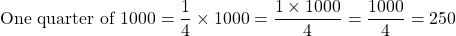Example Two

One half of what number is 250?

Let `x` be the unknown number. Set up the equation as follows and then solve for `x`.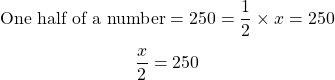What number divided by 2 gives 250. It is 500. Half of 500 gives 250.Pixabay Image Source

## Multiplying Fractions Case

When it comes fractions and multiplying fractions in particular the keyword of means multiply. Here are a few examples.

Example One

What is one half of 200?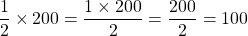Example Two

What is four sevenths of 2100?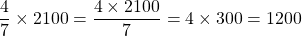Example Three

You can take a fraction of a fraction. What is one third of seven tenths?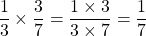Pixabay Image Source

## Percentages

Percentages deal with parts of a whole. One percent is one of each 100 or 1/100 as a fraction. In the following examples the percentages are converted into fractions before multiplying fractions.

Example One

What is 10% of 300?

Ten percent is 10 out of 100 which can be reduced to 1 out of 10. This one tenth is multiplied with 300 to obtain 30.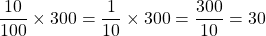Example Two

A country's tax rate on purchases is 8%. If Maria's purchases before tax comes to \$230, how much tax does Maria pay?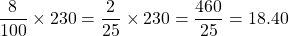Maria would pay \$18.40 in taxes on top of the \$230 purchase.

Example Three

Percentages can be above 100% sometimes. What is 550% of 20?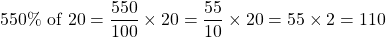Pixabay Image Source

## Sample Of A Whole

The keyword of can be applied to introductory data management and introductory statistics.

A population is a group you want to get information from. It is often not practical to obtain information from every member from a population. It takes time and can cost a lot of money to do so. Taking a sample of the population involves taking a portion of the population to collect data with less time and less cost. A sample's size is less than the population.

When a sample is taken, the sample is part of the greater population. Information can be gathered from the sample to estimate characteristics or properties from the larger population.

Reference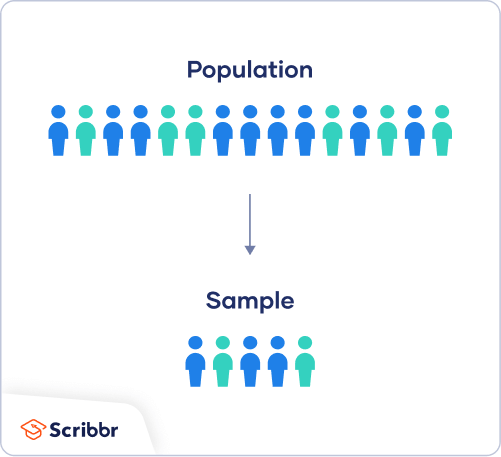Image From Scribbr

Posted with STEMGeeks

Sort:

Uno bueno hombre, este tipo de información son necesarios, gracias por este aporte.
!1UP#### You have received a 1UP from @latino.romano!

The following @oneup-cartel family members will soon upvote your post:
`@stem-curator`
And they will bring !PIZZA 🍕